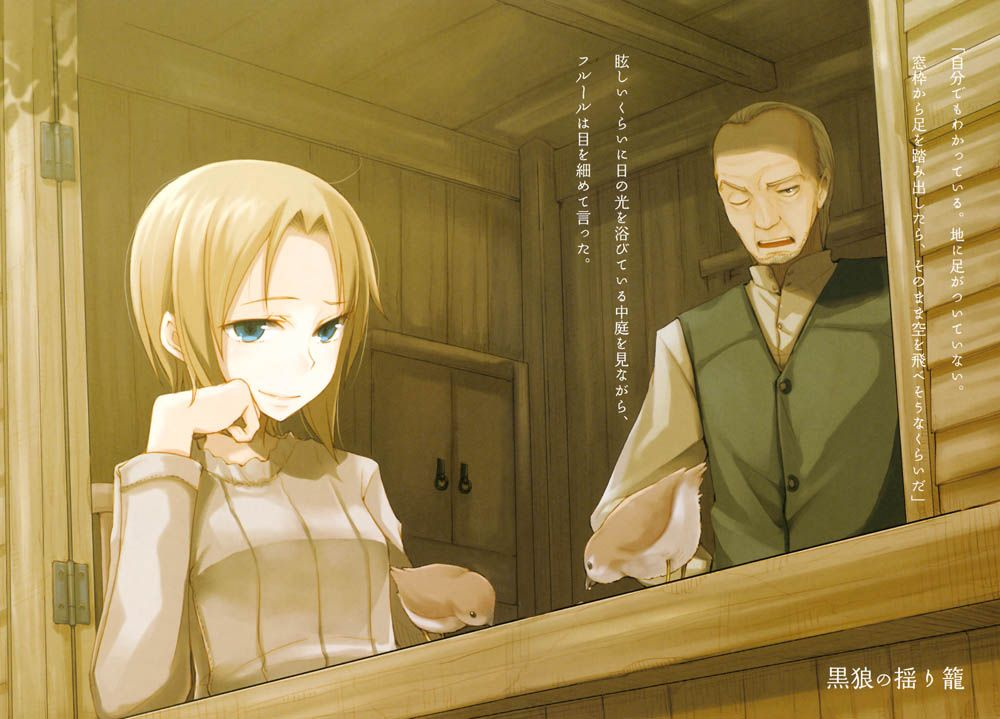# 线性代数笔记（8）——求解非齐次线性方程组（续）## 线性代数笔记（8）——求解非齐次线性方程组（续）

Contents

### 复习

$$A \stackrel{\text{行变换}}{\longrightarrow} U \stackrel{\text{行变换}}{\longrightarrow} U_0 \stackrel{\text{列变换}}{\longrightarrow} \begin{pmatrix} I_r & F \\ 0 & 0 \end{pmatrix}$$

### 求解非齐次线性方程组

#### 解的一般性讨论

1. $r = n = m \Rightarrow \mathbf{x} = A^{-1}\mathbf{b}$（有唯一解）。
2. $r = n < m$，$A \mathbf{x} = \mathbf{0}$ 解的情况：只有零解；此时 $A \mathbf{x} = \mathbf{b}$ 的解：无解有唯一解
3. $r = m < n$，$A$ 主元个数为 $m$，此时自由变量个数为 $n - m$，故此时 $A \mathbf{x} = \mathbf{b}$ 的解：无穷多解
4. $r < m, r < n$，无解无穷多解

#### 一般矩阵的左右逆

1. 若 $A$ 列满秩，则 $A$ 有左逆，经初等行变换，$\exists$ 可逆阵 $E$ 使得 $EA = U_0 = \begin{pmatrix}I_n \\ 0 \end{pmatrix}$，故$A$ 有左逆 $\begin{pmatrix}I_n & 0\end{pmatrix}E$，$\begin{pmatrix}I_n & 0\end{pmatrix}E \cdot A = I_n$。
2. 若 $A$ 行满秩，则 $A$ 有右逆，经初等列变换，$\exists$ 可逆阵 $P$ 使得 $AP = U_0 = \begin{pmatrix}I_m & 0\end{pmatrix}$，故$A$ 有右逆 $P \begin{pmatrix}I_m \\ 0 \end{pmatrix}$，$A \cdot P \begin{pmatrix}I_m \\ 0 \end{pmatrix}= I_m$。